### IMO Shortlist 1999 problem C2

Kvaliteta:
Avg: 0,0
Težina:
Avg: 6,0
If a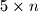$5 \times n$ rectangle can be tiled using$n$ pieces like those shown in the diagram, prove that$n$ is even. Show that there are more than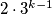$2 \cdot 3^{k-1}$ ways to file a fixed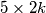$5 \times 2k$ rectangle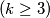$(k \geq 3)$ with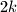$2k$ pieces. (symmetric constructions are supposed to be different.)
Izvor: Međunarodna matematička olimpijada, shortlist 1999